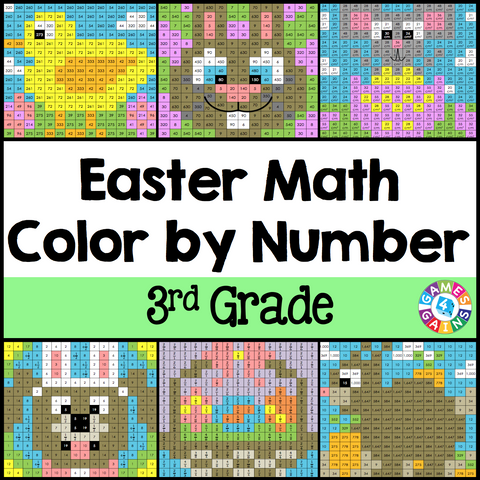## Easter Math Color-by-Number - 3rd Grade

• \$480

Easter Math Color-by-Number set comes with 6 Easter Math color-by-number activities for reviewing 3rd grade math skills. This Easter Math math set is perfect to use in centers, in small groups, or with the whole class!

Included with this Easter Math Math Color-by-Number set:
Instructions
6 color by number worksheets
6 student problem worksheets
6 teacher answer sheets

Here are the skills covered by these Easter Math math color-by-number activities:

• Determining the unknown in multiplication by multiples of 10 equations
• Determining the next number in an addition or subtraction pattern
• Comparing fractions with the same denominator or same numerator
• Solving word problems for metric mass and capacity (g, kg, mL, L)
• Calculating the perimeter and area of rectangles on a grid
• Solving problems using line plots (with units by 1/4 and 1/2)

Want to learn more about what you'll get? Check out the preview to see a sample of the actual pages.